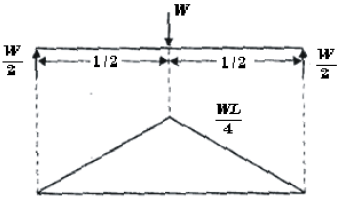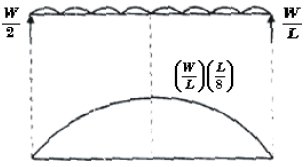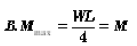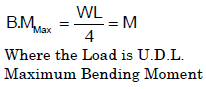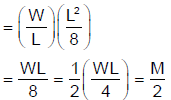Courses

# Bending Moment And Shear Force Diagram - MCQ Test 1

## 20 Questions MCQ Test Mock Test Series - Mechanical Engineering (ME) for GATE 2020 | Bending Moment And Shear Force Diagram - MCQ Test 1

Description
This mock test of Bending Moment And Shear Force Diagram - MCQ Test 1 for Mechanical Engineering helps you for every Mechanical Engineering entrance exam. This contains 20 Multiple Choice Questions for Mechanical Engineering Bending Moment And Shear Force Diagram - MCQ Test 1 (mcq) to study with solutions a complete question bank. The solved questions answers in this Bending Moment And Shear Force Diagram - MCQ Test 1 quiz give you a good mix of easy questions and tough questions. Mechanical Engineering students definitely take this Bending Moment And Shear Force Diagram - MCQ Test 1 exercise for a better result in the exam. You can find other Bending Moment And Shear Force Diagram - MCQ Test 1 extra questions, long questions & short questions for Mechanical Engineering on EduRev as well by searching above.
QUESTION: 1

Solution:
QUESTION: 2

### Two identical cantilever beams are supported as shown, with their free ends in contact through a rigid roller. After the load P is applied, the free ends will have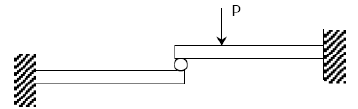Solution:

As it is rigid roller, deflection must be same, because after deflection they also will be in contact. But slope unequal.

QUESTION: 3

### A mass less beam has a loading pattern as shown in the figure. The beam is of rectangular cross -section with a width of 30 mm and height of 100 mm.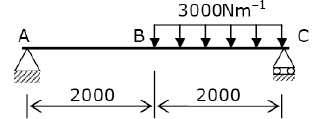The maximum bending moment occurs at

Solution: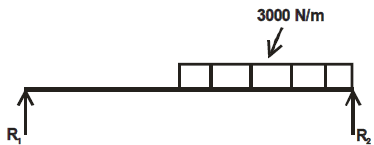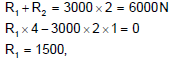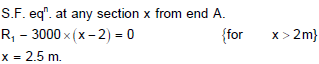QUESTION: 4

The shapes of the bending moment diagram for a uniform cantilever beam carrying a uniformly distributed load over its length is:

Solution: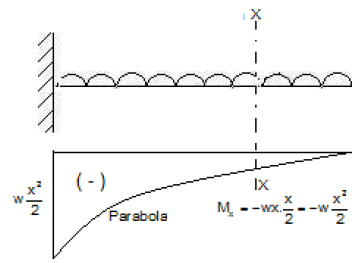QUESTION: 5

Solve the problems and choose correct answers A steel beam of breadth 120 mm and height 750 mm is loaded as shown in the figure. Assume Esteel= 200 GPa.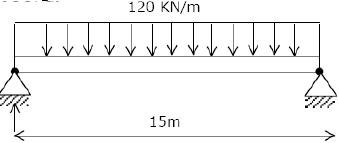The beam is subjected to a maximum bending moment of

Solution: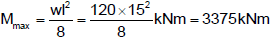QUESTION: 6

A simply supported beam of span length 6m and 75mm diameter carries a uniformly distributed load of 1.5 kN/m

What is the maximum value of bending moment?

Solution: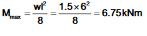QUESTION: 7

A simply supported beam is subjected to a distributed loading as shown in the diagram given below:

What is the maximum shear force in the beam?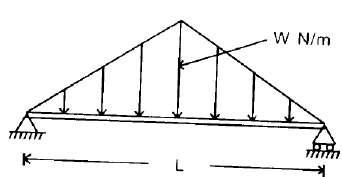Solution: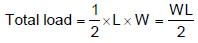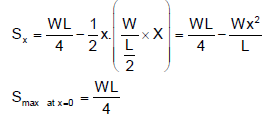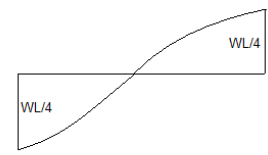QUESTION: 8

A lever is supported on two hinges at A and C. It carries a force of 3 kN as shown in the above figure. The bending moment at B will be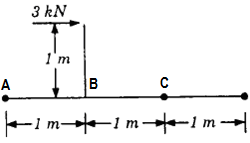Solution:
QUESTION: 9

The bending moment (M) is constant over a length segment (I) of a beam. The shearing force will also be constant over this length and is given by

Solution:

QUESTION: 10

Consider the following statements:

If at a section distant from one of the ends of the beam, M represents the bending moment. V the shear force and w the intensity of loading, then

1. dM/dx = V

2. dV/dx = w

3. dw/dx = y

(the deflection of the beam at the section)

Select the correct answer using the codes given below:

Solution:
QUESTION: 11

If the shear force acting at every section of a beam is of the same magnitude and of the same direction then it represents a

Solution:
QUESTION: 12

The shearing force diagram for a beam is shown in the above figure. The bending moment diagram is represented by which one of the following?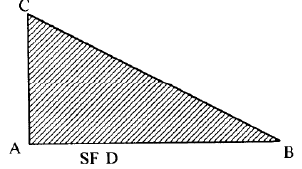Solution:

Uniformly distributed load on cantilever beam.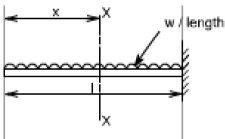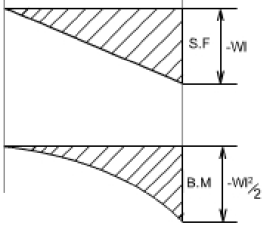QUESTION: 13

A vertical hanging bar of length L and weighing w N/ unit length carries a load W at the bottom. The tensile force in the bar at a distance Y from the support will be given by

Solution:
QUESTION: 14

Assertion (A): If the bending moment along the length of a beam is constant, then the beam cross section will not experience any shear stress.

Reason (R): The shear force acting on the beam will be zero everywhere along the length.

Solution:
QUESTION: 15

What is the bending moment at end supports of a simply supported beam?

Solution:

At the end supports, the moment (couple) developed is zero, because there is no distance to take the perpendicular acting load. As the distance is zero, the moment is obviously zero.

QUESTION: 16

If a beam is subjected to a constant bending moment along its length, then the shear force will

Solution:
QUESTION: 17

A simply supported beam has equal over-hanging lengths and carries equal concentrated loads P at ends. Bending moment over the length between the supports

Solution:
QUESTION: 18

Which one of the following portions of the loaded beam shown in the given figure is subjected to pure bending?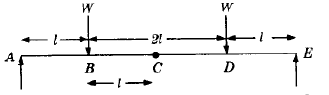Solution:

Pure bending takes place in the section between two weights W

QUESTION: 19

At the point of contraflexure, the value of bending moment is____

Solution:
QUESTION: 20

A freely supported beam at its ends carries a central concentrated load, and maximum bending moment is M. If the same load be uniformly distributed over the beam length, then what is the maximum bending moment?

Solution: